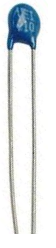﻿ Thermistor Resistance- Explained

# Thermistor Resistance- ExplainedIn this article, we go over how the resistance of thermistors change in response to the temperature they are exposed to.

Thermistors are specialized resistors whose resistance values change in accordance to the temperature they are exposed to. Depending on the amount of heat or cold a thermistor is exposed to determines the amount of resistance they will offer in a circuit.

There are 2 main types of thermistors: negative temperature coefficient (NTC) thermistors and positive temperature coefficient (PTC) thermistors.

NTC and PTC thermistors both change in accordance to the temperature they are exposed to, but they do so in opposite manners.

### NTC Thermistor Resistance

Negative temperature coefficient (NTC) thermistors are thermistors whose resistance value decreases when the temperature they are exposed to increases.

It's an inverse relationship. As temperature goes up, resistance goes down. As temperature goes down, resistance goes up.

Below is a chart of many different types of NTC thermistors of different rated resistance values.These are NTC thermistors of different rated resistance values. You can see that when temperature is low, resistance is high. As temperature increases, as we go from left to right, the resistance of the thermistors gradually decrease. This is how NTC thermistors operate. Temperature and resistance have an inverse relationship.

### PTC Thermistor Resistance

Positive temperature coefficient (PTC) thermistors are thermistors whose resistance value increases when the temperature they are exposed to increases.

It's a direct relationship. As temperature goes up, resistance goes up. As temperature goes down, resistance goes down.

Below is a chart of many different types of PTC thermistors of different rated resistance values.These are PTC thermistors of different rated resistance values. You can see that when temperature is low, resistance is low. As temperature increases, as we go from left to right, the resistance of the thermistors gradually increase. This is how PTC thermistors operate. Temperature and resistance have a direct relationship.

Related Resources

How to Build Simple Thermistor Circuits

How to Test a Thermistor

How to Build a Heat Alarm Circuit